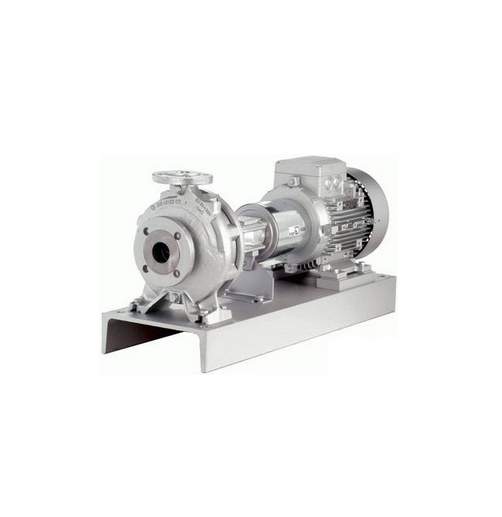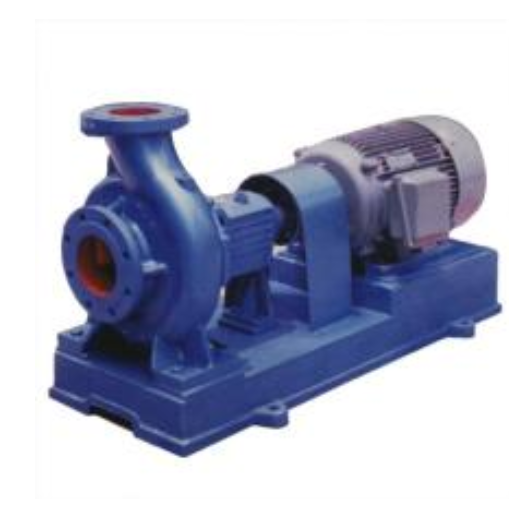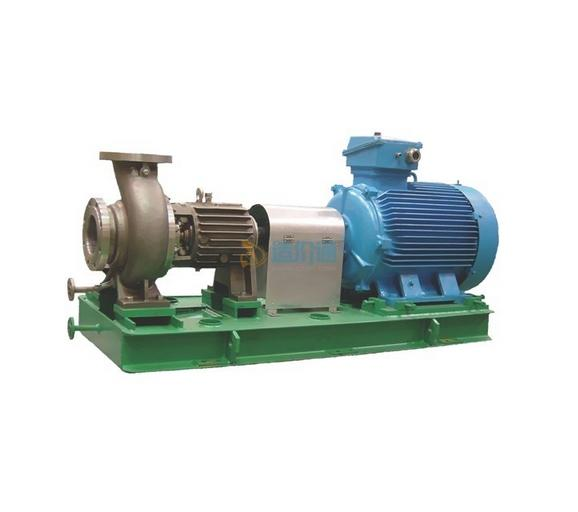## 广东市场价分类

2016年5月之前为含税价格，2016年5月营改增后显示的为除税价格
除税价)

#### 太阳能循环泵

q=3.6L/S h=20m p=1.5KW

q=3.6L/S h=20m p=1.5KW• 市场价
• 建议价
• 品牌 宇唐环保
宇唐环保
• 单位
• 税率13%广州宇唐环保设备有限公司

#### 冷冻水泵

H=34.5m Q=400m3 N=75KW

H=34.5m Q=400m3 N=75KW• 市场价
• 建议价
• 品牌 广一
广一
• 单位
• 税率13%广州广一泵业有限公司

#### 冷冻水泵

H=35m Q=200m3 N=30KW

H=35m Q=200m3 N=30KW• 市场价
• 建议价
• 品牌 广一
广一
• 单位
• 税率13%广州广一泵业有限公司

#### 冷却水泵

H=26m Q=480m3 N=55KW

H=26m Q=480m3 N=55KW• 市场价
• 建议价
• 品牌 广一
广一
• 单位
• 税率13%广州广一泵业有限公司

#### 冷却水泵

P>40 H=26m Q=480m3 N=55KW]

P>40 H=26m Q=480m3 N=55KW]• 市场价
• 建议价
• 品牌 广一
广一
• 单位
• 税率13%广州广一泵业有限公司

#### 热水循环泵• 市场价
• 建议价
• 品牌 荏原
荏原
• 单位
• 税率13%荏原机械（中国）有限公司广州办事处

#### 热水循环泵• 市场价
• 建议价
• 品牌 荏原
荏原
• 单位
• 税率13%荏原机械（中国）有限公司广州办事处

#### 太阳能循环泵

q=3.6L/S h=15m p=4.0KW

q=3.6L/S h=15m p=4.0KW• 市场价
• 建议价
• 品牌 白云泵业
白云泵业
• 单位
• 税率13%广州白云泵业有限公司佛山分公司

#### 太阳能循环泵

q=3.6L/S h=20m p=1.5KW

q=3.6L/S h=20m p=1.5KW• 市场价
• 建议价
• 品牌 白云泵业
白云泵业
• 单位
• 税率13%广州白云泵业有限公司佛山分公司

#### 循环泵

7.5KW 扬程 8米 207m3/h

7.5KW 扬程 8米 207m3/h• 市场价
• 建议价
• 品牌
• 单位
• 税率13%惠州童欣泳池设备有限公司

#### 循环水泵(带毛发收集器)

3.5HP 扬程 9m 38m3/h

3.5HP 扬程 9m 38m3/h• 市场价
• 建议价
• 品牌
• 单位
• 税率13%惠州童欣泳池设备有限公司

#### 热水循环泵

BYR40-125(I)A 流量 8m3/h功率 1.1KW

BYR40-125(I)A 流量 8m3/h功率 1.1KW• 市场价
• 建议价
• 品牌 白云泵业
白云泵业
• 单位
• 税率13%广州白云泵业有限公司佛山分公司

#### 太阳能循环泵

BYR50-125 流量 13m3/h 功率 1.5KW

BYR50-125 流量 13m3/h 功率 1.5KW• 市场价
• 建议价
• 品牌 白云泵业
白云泵业
• 单位
• 税率13%广州白云泵业有限公司佛山分公司

#### 循环泵

KQL250/300-55/4 Q=320m3/h H=26m N=55kW 冷冻

KQL250/300-55/4 Q=320m3/h H=26m N=55kW 冷冻• 市场价
• 建议价
• 品牌
• 单位
• 税率13%佛山市禅城区一龙泵业有限公司

#### 循环泵

Q=24m3/h H=15.5m N=2.2KW 3HP

Q=24m3/h H=15.5m N=2.2KW 3HP• 市场价
• 建议价
• 品牌
• 单位
• 税率13%广州市碧浪游泳池设备有限公司

#### 循环泵

Q=40m3/h H=13m N=4KW

Q=40m3/h H=13m N=4KW• 市场价
• 建议价
• 品牌
• 单位
• 税率13%广州德奥环保设备有限公司

#### 循环泵

Q=60m3/h H=13m N=5.5KW

Q=60m3/h H=13m N=5.5KW• 市场价
• 建议价
• 品牌
• 单位
• 税率13%东莞市小水滴水处理科技有限公司

#### 冷却水循环泵

KQL-300/315B-55/4 Q=400m3/h H=19m N=55kW 冷冻

KQL-300/315B-55/4 Q=400m3/h H=19m N=55kW 冷冻• 市场价
• 建议价
• 品牌
• 单位
• 税率13%佛山市禅城区一龙泵业有限公司

#### 热泵制热循环泵

PH-1500QH N=1.75KW Q=266L/min H=15m

PH-1500QH N=1.75KW Q=266L/min H=15m• 市场价
• 建议价
• 品牌
• 单位
• 税率13%广州安德斯机电设备有限公司

#### 热泵循环泵

WK12-7 Q=12m3/h H=35m N=2.2kw

WK12-7 Q=12m3/h H=35m N=2.2kw• 市场价
• 建议价
• 品牌 冠申
冠申
• 单位
• 税率13%深圳市冠申泵业有限公司

1 2 3 4 5 6 7 8 9 10 跳转到第 GO